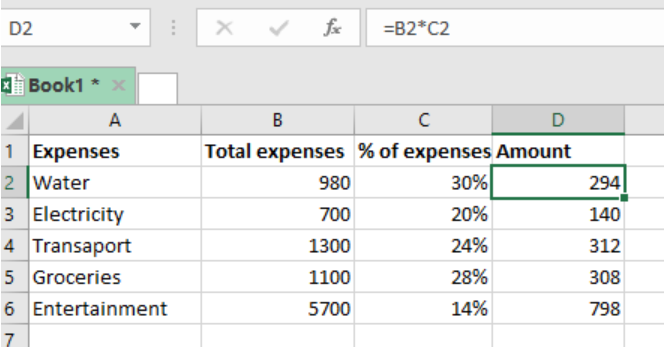Get instant live expert help with Excel or Google Sheets“My Excelchat expert helped me in less than 20 minutes, saving me what would have been 5 hours of work!”

#### Post your problem and you’ll get Expert help in seconds.

Your message must be at least 40 characters
Our professional Expert are available now. Your privacy is guaranteed.

# Get amount with percentage

You can use a simple formula to calculate the amount that is being represented by a given percentage if you are supplied with a total and a percentage that represents some amount of the total. This article provides a step-by-step guide on how to get amount with percentage with excel.Figure 1 – Quick Explanation

## Syntax of the formula

`=total * percentage`

## Understanding the formula

For us to calculate the amount, we need to simply multiply the ‘totals’ cell by the ‘percentage’ cell. The result we get shall reflect the amount spent on that particular item.Figure 2 – Final result

When working, we need to format the percentage column to reflect the percentages. This will simplify your work of multiplying the cell references. In the example above, we have calculated the amount of each expenses. The results are in column D.  In cell D2 for example, we have used the formula =B2*C2. This has given us the amount of the amount of the water expense.

To get the amount for the other cells, we simply copy down the formula across the other cells.

## Instant Connection to an Expert through our Excelchat Service

Most of the time, the problem you will need to solve will be more complex than a simple application of a formula or function. If you want to save hours of research and frustration, try our live Excelchat service! Our Excel Experts are available 24/7 to answer any Excel question you may have. We guarantee a connection within 30 seconds and a customized solution within 20 minutes.

### Did this post not answer your question? Get a solution from connecting with the expert.Another blog reader asked this question today on Excelchat:## Subscribe to Excelchat.coAnother blog reader asked this question today on Excelchat: